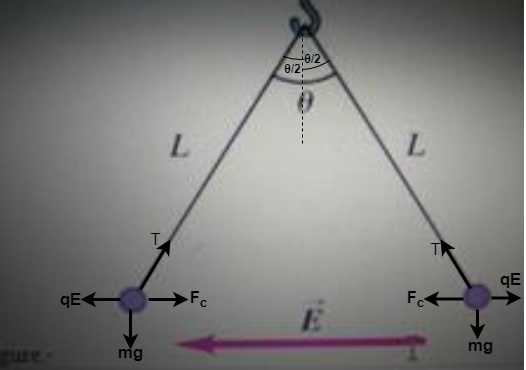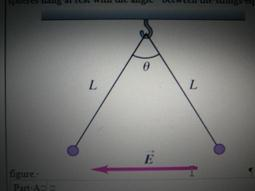# Problem: Two tiny spheres of mass m = 3.00 mg carry charges of equal magnitude, 72.0nC, but opposite sign. They are tied to the same ceiling hook by light strings of length 0.530m. When a horizontal uniform electric field E that is directed to the left is turned on, the spheres hang at rest with the angle θ between the strings equal to 50.0 degrees in the figure below.What's the magnitude E of the field and which ball (left or right) has a positive charge?

###### FREE Expert Solution

Let's visualize the problem in the figure below:From this figure, let's consider the force on a positive charge in the vertical direction.

The forces balance at equilibrium.

94% (52 ratings)###### Problem Details

Two tiny spheres of mass m = 3.00 mg carry charges of equal magnitude, 72.0nC, but opposite sign. They are tied to the same ceiling hook by light strings of length 0.530m. When a horizontal uniform electric field E that is directed to the left is turned on, the spheres hang at rest with the angle θ between the strings equal to 50.0 degrees in the figure below.What's the magnitude E of the field and which ball (left or right) has a positive charge?